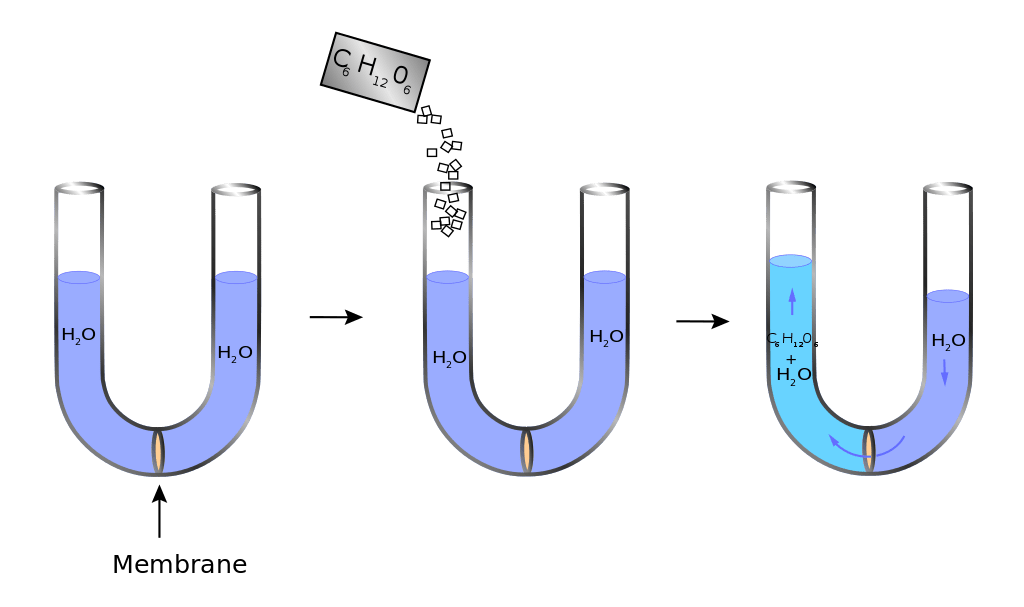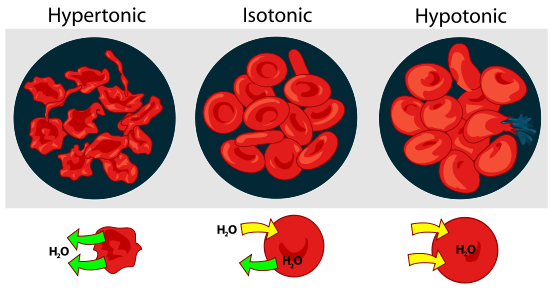# Difference Between Osmolarity and Tonicity

## Main Difference – Osmolarity vs Tonicity

The terms Osmolarity and Tonicity are closely related to each other. They refer to the concept of concentrations of solutions and how they affect the osmotic pressure. However, the way they express their effects is different from each other. Both terms relate to situations where solutions are separated by permeable membranes where osmosis takes place. Osmolarity takes into consideration all the molecules present in the solution for its collective effect whereas, tonicity only considers the concentration of the non-permeable solute molecules within the solution. Therefore, it can be said that tonicity is a measure of the external environment, whereas osmolarity concerns the given solution itself. This is the main difference between osmolarity and tonicity.

## What is Osmolarity

Osmolarity is a way of expressing the concentration of a solution by means of the number of osmoles present in the solution rather than the well-known way of considering the number of moles in a solution which is referred to as ’molarity’. Therefore instead of the unit mol/L like in the case of molarity, osmolarity uses the units osmoles of solute per litre or osmol/L. In many cases, the value for osmolarity is similar to the value of molarity, however, in the case of water-soluble compounds, the osmolarity becomes greater than the solution’s molarity. This is because, osmolarity involves the total amount of solutes present whereas the molarity involves the concentration of the compound as a whole. For instance; NaCl once dissolved in water separates into its ions as Na+ and Cl. If  NaCl had a concentration of 2 mol/L at the start, upon the dissolution in water, its molarity remains 2 mol/L but its osmolarity increases to 4 osmol/L, due to the consideration of the total number of solutes in solution now, which are the separated ions. (2 osmol/L of Na+ and 2 osmol/L of Cl).

Depending on the characteristics of the surrounding environment, the osmolarity of a given solution can take three different forms. Isosmotic, hyperosmotic and hypoosmotic. When the osmotic pressure of the given solution is equal to its surrounding, it is called an isosmotic solution. A solution is hyperosmotic if its osmotic pressure is higher than its surrounding. Similarly, a solution is called hypoosmotic if its osmotic pressure is lower than its surroundings.## What is Tonicity

Unlike osmolarity, tonicity is influenced by the solutes that do not cross the membrane. Therefore, it is a measure of the osmotic pressure gradient and not the osmotic pressure itself. The concept of tonicity is helpful in describing the reactions and behaviour of a cell immersed in different strengths of concentrations. If a cell is placed in an isotonic solution, the concentration of the solution is similar to the concentration of the cellular contents. Therefore, there will not be an influx or efflux of the solvent molecules through the semi-permeable membrane. However, if the cell is placed in a solution which is hypertonic, the concentration of the solution is higher than the concentration of the cellular contents. Therefore the solvent molecules (in this case its water), will flow out of the cell till the concentrations are equal on either side of the membrane, causing it to shrink. Similarly, if the cell was placed in a hypotonic solution, as the concentration of the solution is lower than that of the cellular contents, there will be an influx of water into the cell, causing the cell to swell and burst off eventually.Effect of different solutions on blood cells

## Difference Between Osmolarity and Tonicity

### Definition

Osmolarity is a measure of the osmotic pressure of a given solution.

Tonicity is a measure of the osmotic pressure gradient between two solutions separated by a permeable membrane.

### Units of Measurement

Osmolarity is measured in osmol/L.

Tonicity is related to concentration measurements and is expressed in terms of mol/L.

### Defining Concentration

Osmolarity takes into consideration the total number of solutes that penetrates and do not penetrate the membrane.

Tonicity takes into account the concentration relating to the non-permeable solutes.

### Effect of Surrounding

Osmolarity often represents the analysis of a given solution.

Tonicity is used as a measure of the external environment.Image Courtesy:

“Osmotic pressure on blood cells diagram” by LadyofHats. (Public Domain) via Commons

“Osmosis diagram” by KDS4444 – Own work. (CC0) via Common